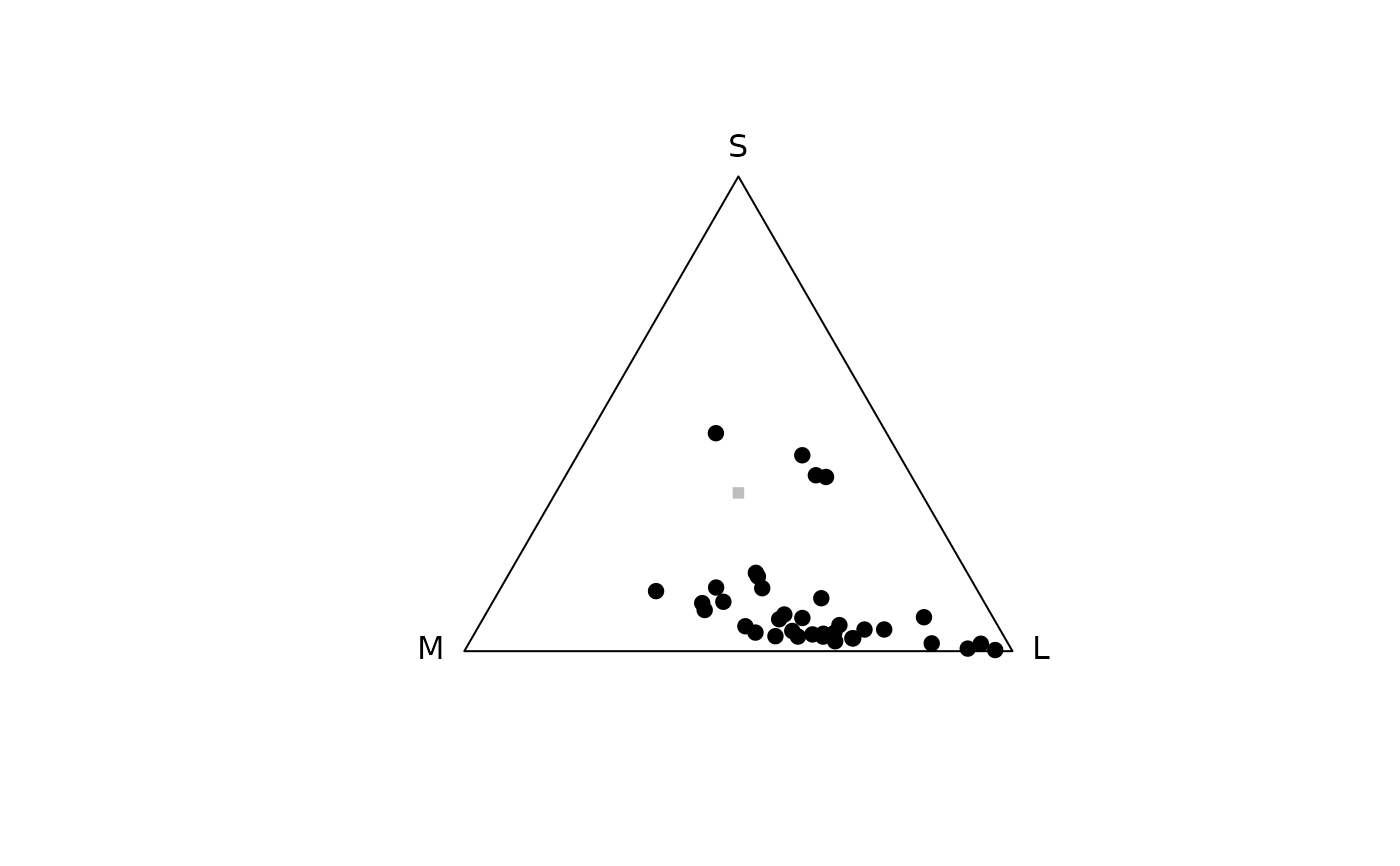Models reflectance spectra in a colorspace. For information on plotting arguments and graphical parameters, see plot.colspace().

colspace(
vismodeldata,
space = c("auto", "di", "tri", "tcs", "hexagon", "coc", "categorical", "ciexyz",
"cielab", "cielch", "segment"),
qcatch = NULL,
...
)

## Arguments

vismodeldata

(required) quantum catch color data. Can be either the result from vismodel() or independently calculated data (in the form of a data frame with columns representing quantum catches).

space

Which colorspace/model to use. Options are:

• auto: if data is a result from vismodel(), applies di, tri or tcs if input visual model had two, three or four cones, respectively.

• di: dichromatic colourspace. See dispace() for details. (plotting arguments)

• tri: trichromatic colourspace (i.e. Maxwell triangle). See trispace() for details. (plotting arguments)

• tcs: tetrahedral colourspace. See tcspace() for details. (plotting arguments)

• hexagon: the trichromatic colour-hexagon of Chittka (1992). See hexagon() for details. (plotting arguments)

• coc: the trichromatic colour-opponent-coding model of Backhaus (1991). See coc() for details. (plotting arguments)

• categorical: the tetrachromatic categorical fly-model of Troje (1993). See categorical() for details. (plotting arguments)

• ciexyz: CIEXYZ space. See cie() for details. (plotting arguments)

• cielab: CIELAB space. See cie() for details. (plotting arguments)

• cielch: CIELCh space. See cie() for details. (plotting arguments)

• segment: segment analysis of Endler (1990). See segspace() for details. (plotting arguments)

qcatch

Which quantal catch metric is being inputted. Only used when input data is NOT an output from vismodel(). Must be Qi, fi or Ei.

...

additional arguments passed to cie() for non vismodel() data.

## Author

Rafael Maia rm72@zips.uakron.edu

Thomas White thomas.white026@gmail.com

## Examples

data(flowers)

# Model a dichromat viewer in a segment colourspace
vis.flowers <- vismodel(flowers, visual = "canis")
di.flowers <- colspace(vis.flowers, space = "di")

# Model a honeybee viewer in the colour hexagon
vis.flowers <- vismodel(flowers,
visual = "apis", qcatch = "Ei", relative = FALSE,
vonkries = TRUE, achromatic = "l", bkg = "green"
)
hex.flowers <- colspace(vis.flowers, space = "hexagon")

# Model a trichromat (the honeybee) in a Maxwell triangle
vis.flowers <- vismodel(flowers, visual = "apis")
tri.flowers <- colspace(vis.flowers, space = "tri")
plot(tri.flowers)# Model a tetrachromat (the Blue Tit) in a tetrahedral colourspace
vis.flowers <- vismodel(flowers, visual = "bluetit")
tcs.flowers <- colspace(vis.flowers, space = "tcs")

# Model a housefly in the 'categorical' colourspace
vis.flowers <- vismodel(flowers, visual = "musca", achro = "md.r1")
cat.flowers <- colspace(vis.flowers, space = "categorical")